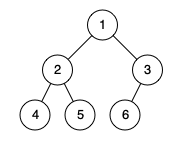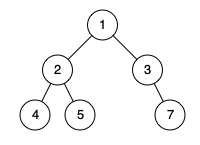0958. Check Completeness of a Binary Tree

# 958. Check Completeness of a Binary Tree#

## 题目 #

Given the `root` of a binary tree, determine if it is a complete binary tree.

In a  complete binary tree, every level, except possibly the last, is completely filled, and all nodes in the last level are as far left as possible. It can have between `1` and `2h` nodes inclusive at the last level `h`.

Example 1:``````Input: root = [1,2,3,4,5,6]
Output: true
Explanation: Every level before the last is full (ie. levels with node-values {1} and {2, 3}), and all nodes in the last level ({4, 5, 6}) are as far left as possible.

``````

Example 2:``````Input: root = [1,2,3,4,5,null,7]
Output: false
Explanation: The node with value 7 isn't as far left as possible.

``````

Constraints:

• The number of nodes in the tree is in the range `[1, 100]`.
• `1 <= Node.val <= 1000`

## 解题思路 #

• 这一题是按层序遍历的变种题。
• 判断每个节点的左孩子是否为空。
• 类似的题目，第 102，107，199 题都是按层序遍历的。

## 代码 #

``````package leetcode

import (
"github.com/halfrost/leetcode-go/structures"
)

// TreeNode define
type TreeNode = structures.TreeNode

/**
* Definition for a binary tree node.
* type TreeNode struct {
*     Val int
*     Left *TreeNode
*     Right *TreeNode
* }
*/

func isCompleteTree(root *TreeNode) bool {
queue, found := []*TreeNode{root}, false
for len(queue) > 0 {
node := queue //取出每一层的第一个节点
queue = queue[1:]
if node == nil {
found = true
} else {
if found {
return false // 层序遍历中，两个不为空的节点中出现一个 nil
}
//如果左孩子为nil，则append进去的node.Left为nil
queue = append(queue, node.Left, node.Right)
}
}
return true
}
``````Apr 8, 2023Edit this page